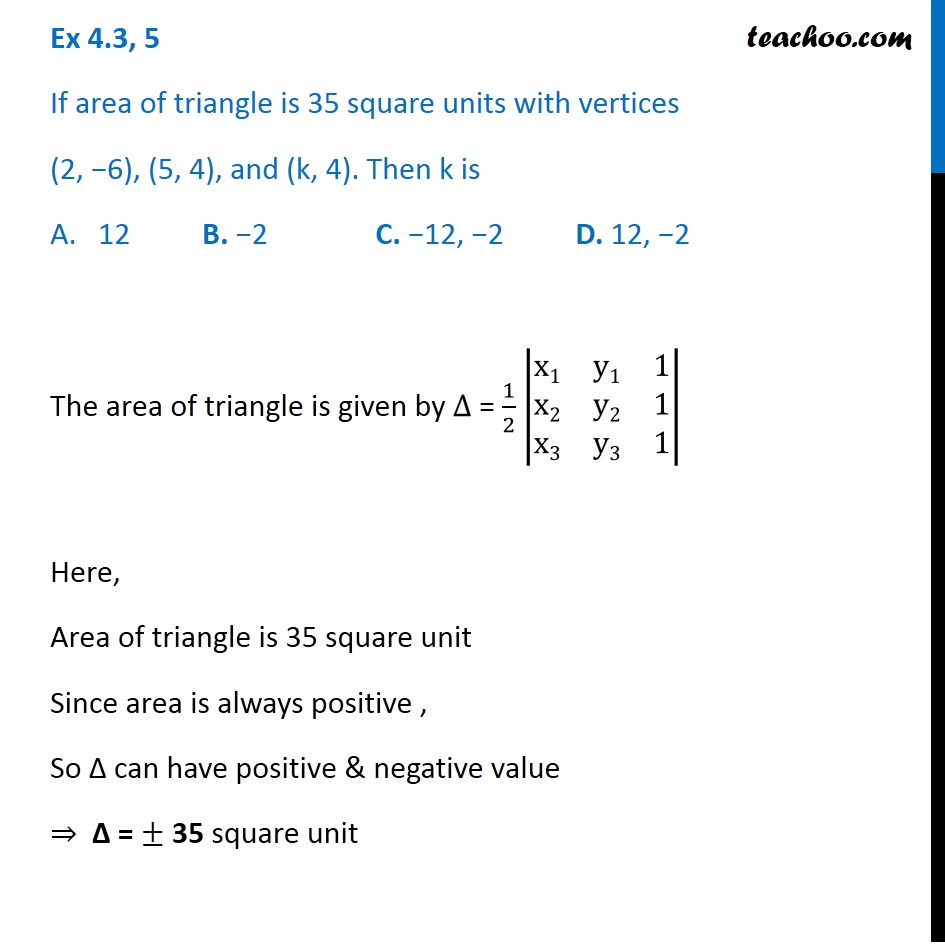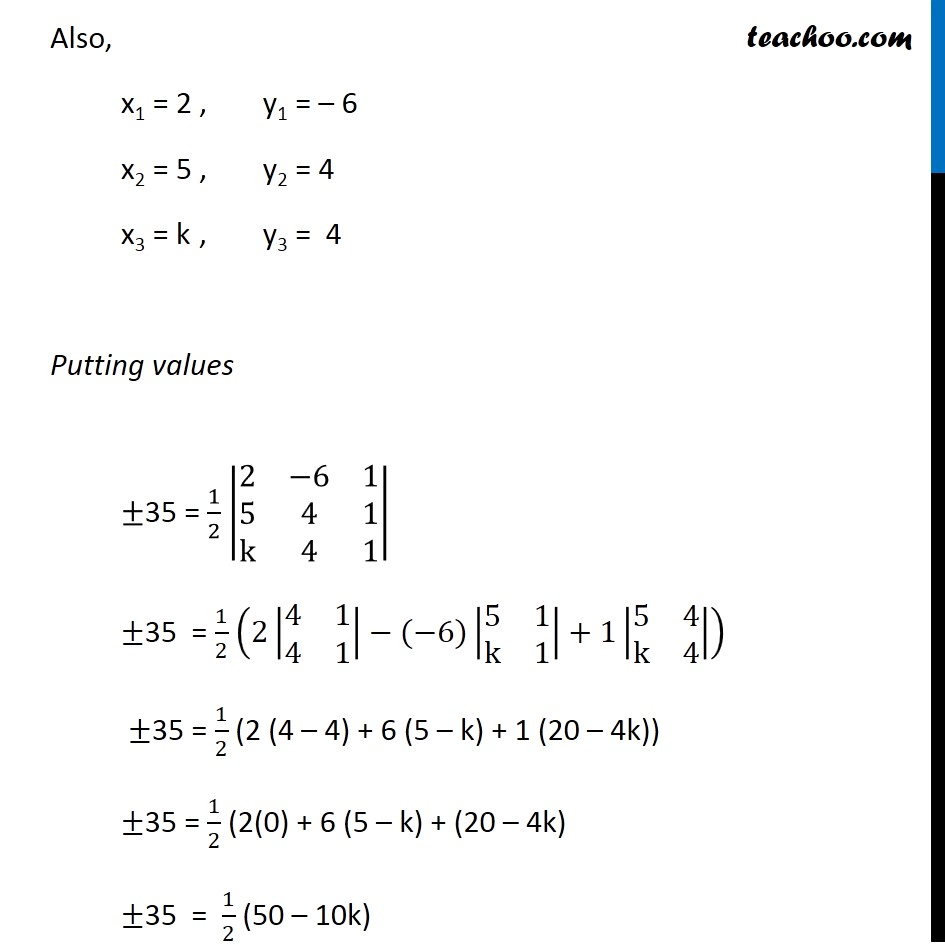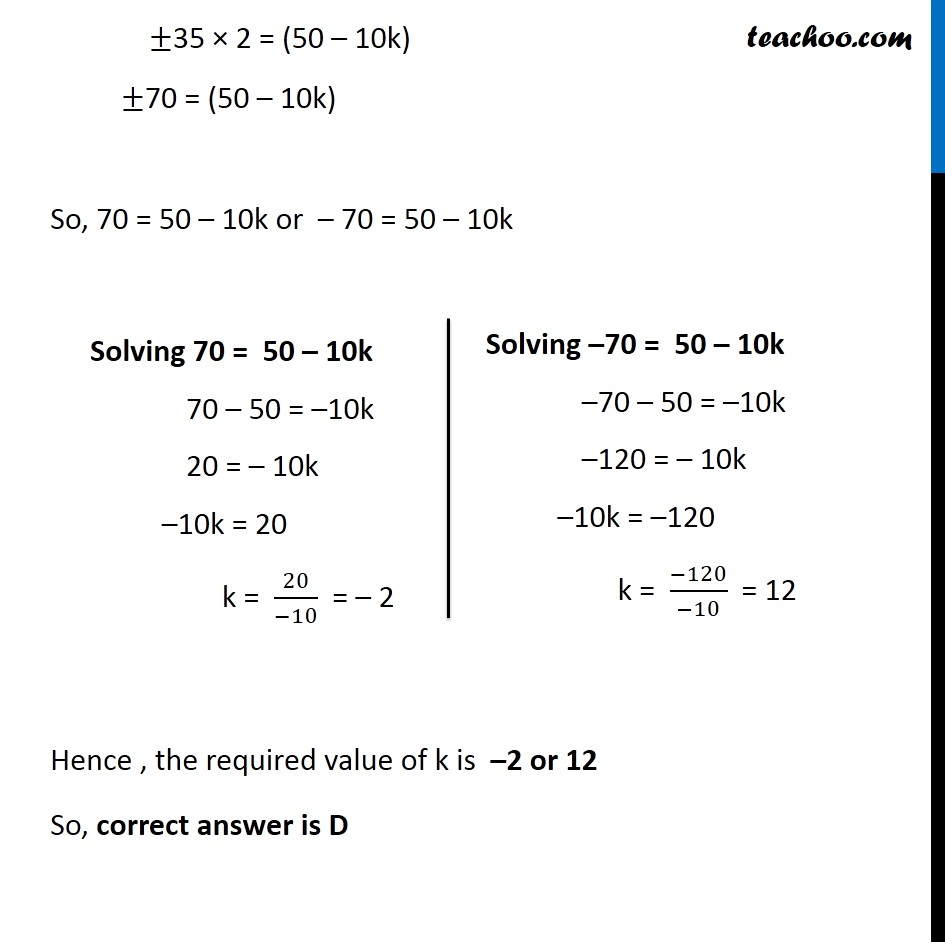Ex 4.2

Chapter 4 Class 12 Determinants
Serial order wiseLearn in your speed, with individual attention - Teachoo Maths 1-on-1 Class

### Transcript

Ex 4.2, 5 If area of triangle is 35 square units with vertices (2, −6), (5, 4), and (k, 4). Then k is 12 B. −2 C. −12, −2 D. 12, −2 The area of triangle is given by ∆ = 1/2 |■8(x1&y1&[email protected]&y2&[email protected]&y3&1)| Here, Area of triangle is 35 square unit Since area is always positive , So ∆ can have positive & negative value ⇒ ∆ = ± 35 square unit Also, x1 = 2 , y1 = – 6 x2 = 5 , y2 = 4 x3 = k , y3 = 4 Putting values ±35 = 1/2 |■8(2&−6&[email protected]&4&[email protected]&4&1)| ±35 = 1/2 (2|■8(4&[email protected]&1)|−(−6)|■8(5&[email protected]&1)|+1|■8(5&[email protected]&4)|) ±35 = 1/2 (2 (4 – 4) + 6 (5 – k) + 1 (20 – 4k)) ±35 = 1/2 (2(0) + 6 (5 – k) + (20 – 4k) ±35 = 1/2 (50 – 10k) ±35 × 2 = (50 – 10k) ±70 = (50 – 10k) So, 70 = 50 – 10k or – 70 = 50 – 10k Hence , the required value of k is –2 or 12 So, correct answer is D Solving 70 = 50 – 10k 70 – 50 = –10k 20 = – 10k –10k = 20 k = 20/(−10) = – 2 Solving –70 = 50 – 10k –70 – 50 = –10k –120 = – 10k –10k = –120 k = (−120)/(−10) = 12